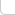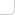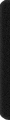# 某岛

… : "…アッカリ～ン . .. . " .. .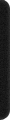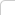July 2, 2019

## EP 01. Defi 合约鉴赏之 Uniswap（WIP）

[latexpage]

### 做市机制 Market Maker Mechanism

Uniswap 最为突出的特性就是她的做市机制（Market Maker Mechanism）足够的简单。与 Bancor 和 P3D 类似，Uniswap 中也没有传统交易所中的 Order Book，直接在合约中用市价交易，通过合约中的储备金来提供流动性。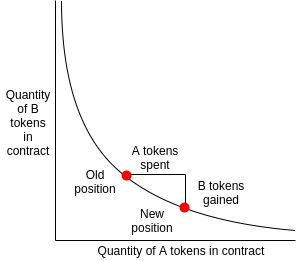– $x$、$y$ 分别是 transcation 执行前合约中储备的 ETH 和 DAI。
– $x’$、$y’$ 分别是 transcation 执行结束之后合约中新的的 ETH 和 DAI。
– $\Delta x$ 为下面的 swap() 方法中传入的 ETH。
– $\Delta y$ 是 swap() 方法兑换出的 DAI。

x*y = k invariant 指的就是：

$$x \times y = k = x’ \times y’ = (x + \Delta x) \times (y + \Delta y)$$

$$Delta y = \frac{k}{(x + \Delta x)} – y$$

eth_pool: uint256
token_pool: uint256

@public
@payable
def ethToTokenSwap():
fee: uint256 = msg.value / 500
invariant: uint256 = self.eth_pool * self.token_pool
new_eth_pool: uint256 = self.eth_pool + msg.value
new_token_pool: uint256 = invariant / (new_eth_pool - fee)
tokens_out: uint256 = self.token_pool - new_token_pool
self.eth_pool = new_eth_pool
self.token_pool = new_token_pool
self.token.transfer(msg.sender, tokens_out


@public
def tokenToEthSwap(tokens_in: uint256):
fee: uint256 = tokens_in / 500
invariant: uint256 = self.eth_pool * self.token_pool
new_token_pool: uint256 = self.token_pool + tokens_in
new_eth_pool: uint256 = self.invariant / (new_token_pool - fee)
eth_out: uint256 = self.eth_pool - new_eth_pool
self.eth_pool = new_eth_pool
self.token_pool = new_token_pool
self.token.transferFrom(msg.sender, self, tokens_out)
send(msg.sender, eth_out)


### 尾声 Epilogue

（TBD）